• 数据结构-二维数组-三角矩阵压缩存储一、什么是三角矩阵前情提要三角矩阵也是属于一类特殊的二维数组矩阵，同样也用压缩的存储方式，能够更好的节约存储空间，二维数组的三角矩阵分为上三角矩阵和下三角矩阵，其实现...
数据结构-二维数组-三角矩阵压缩存储
一、什么是三角矩阵
前情提要
三角矩阵也是属于一类特殊的二维数组矩阵，同样也用压缩的存储方式，能够更好的节约存储空间，二维数组的三角矩阵分为上三角矩阵和下三角矩阵，其实现的原理差不多类似，下面就细细道来。
三角矩阵的特点
此处讨论的三角矩阵的行数和列数是一样的，不妨设都设为

n
$n$。如下所示：
⎡⎣⎢⎢⎢⎢⎢⎢⎢⎢⎢⎢⎢⎢a11a12a22a13a23a33δa14a24a34a44⋯⋯⋯⋯⋱a1n−1a2n−1a3n−1a4n−1⋮an−1n−1a1na2na3na4n⋮an−1nann⎤⎦⎥⎥⎥⎥⎥⎥⎥⎥⎥⎥⎥⎥\begin{bmatrix}
{a_{11}}&{a_{12}}&{a_{13}}&{a_{14}}&\cdots&{a_{1n-1}}&{a_{1n}}\\
{}&{a_{22}}&{a_{23}}&{a_{24}}&\cdots&{a_{2n-1}}&{a_{2n}}\\
{}&{}&{a_{33}}&{a_{34}}&\cdots&{a_{3n-1}}&{a_{3n}}\\
{}&{}&{}&{a_{44}}&\cdots&{a_{4n-1}}&{a_{4n}}\\
{}&{}&\delta&{}&\ddots&\vdots&\vdots\\
{}&{}&{}&{}&{}&{a_{n-1n-1}}&{a_{n-1n}}\\
{}&{}&{}&{}&{}&{}&{a_{nn}}
\end{bmatrix}  如上所示，为上三角矩阵，矩阵的对角线以下的所有元素均为同一常数

δ
$\delta$,或者无效的数据。从上往下逐行的元素总数是比上一行少一个，构成等差数列条件，以下会用的等差数列数学知识。若

δ
$\delta$为常数，则需要在所有元素的最后一个另外加一个元素位置单独存放该数据，毕竟只要是有效数据就需要存储的嘛。对于下三角矩阵有类似的特点，这里放到公式推导里面去介绍。
二、三角矩阵压缩存储
上三角矩阵的存储
如下所示：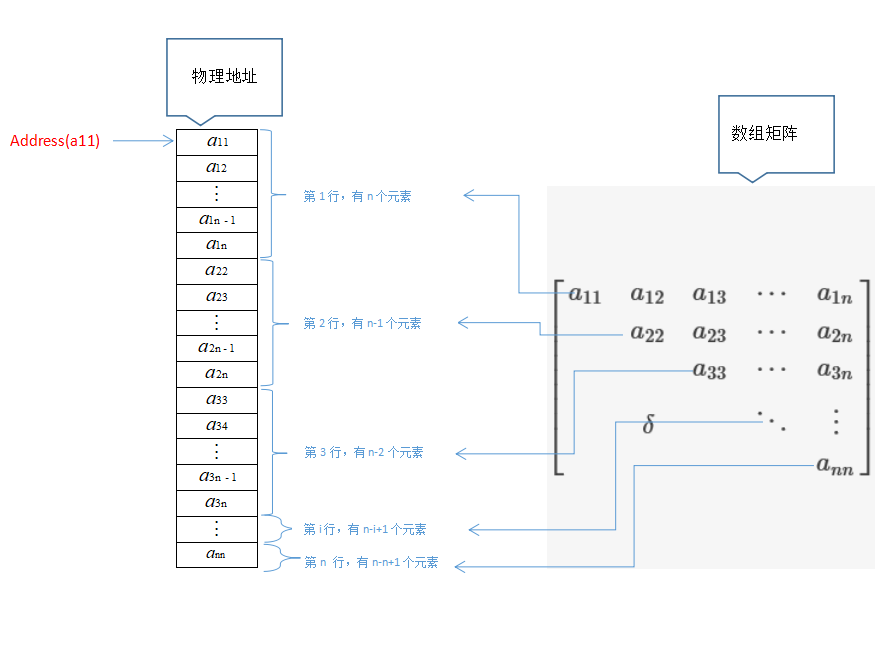对于元素处于上三角区域，即元素

aij
$a_{ij}$，其中

i≤j
$i \le j$，可得如下规律：  第

1
$1$行有n$n$个元素，第

2
$2$行有(n−1)$(n-1)$个元素，第

3
$3$行有(n−2)$(n-2)$个元素，第

i
$i$行有(n−i+1)$(n-i+1)$个元素，…第

n
$n$行有(n−n+1)$(n-n+1)$(即只有

1
$1$个元素)个元素；可得对于元素aij$a_{ij}$，第

(i−1)
$(i-1)$行（即元素

aij
$a_{ij}$前一行）共有：

∑k=1i−1(n−k+1)=(i−1)(2n−i+2)2

\sum_{k=1}^{i-1}(n-k+1)=\frac {(i-1)(2n-i+2)}{2}个元素，元素

aij
${a_{ij}}$,在第i行中是第

(j−i+1)
$(j-i+1)$个元素，规定：每个元素所占的长度为

e
$e$，所以：address(aij)=address(a11)+((i−1)(2n−i+2)2+j−i)eaddress({a_{ij}})=address({a_{11}})+(\frac {(i-1)(2n-i+2)}{2}+j-i)e
对于元素处于下三角区域，即元素

aij
$a_{ij}$，其中

i>j
$i \gt j$，因为下三角区的元素值都一样（如果元素的值有效），则把它放到存储区的最后一个单元,即：

(n+1)n2+1
$\frac {(n+1)n}{2}+1$的位置，可得地址公式：

下三角矩阵的存储
如下所示：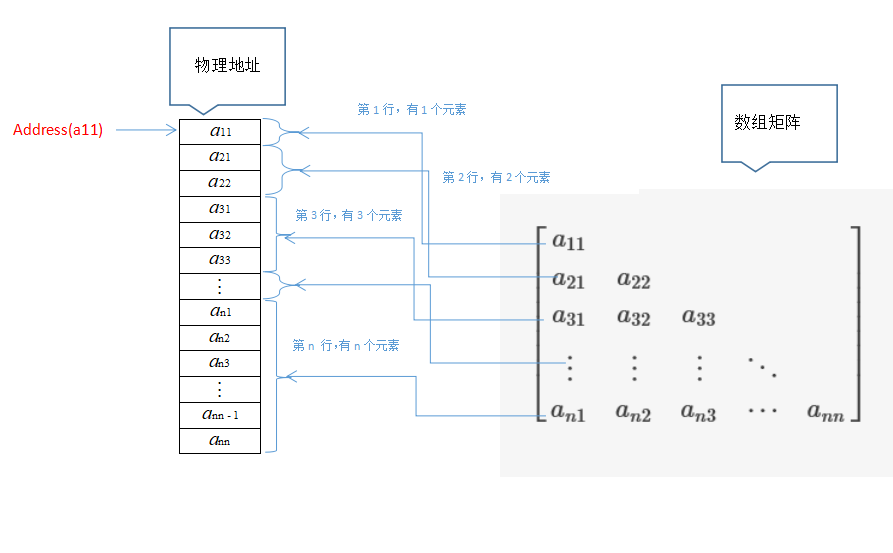对于元素处于下三角区域，即元素

aij
$a_{ij}$，其中

i≥j
$i \ge j$，可得如下规律：  第

1
$1$行有1$1$个元素，第

2
$2$行有2$2$个元素，第

3
$3$行有3$3$个元素，第

i
$i$行有i$i$个元素，…第

n
$n$行有n$n$个元素；可得对于元素

aij
$a_{ij}$，第

(i−1)
$(i-1)$行（即元素

aij
$a_{ij}$前一行）共有：

∑k=1i−1k=(i−1)(1+i−1)2=(i−1)i2

\sum_{k=1}^{i-1}k=\frac {(i-1)(1+i-1)}{2}=\frac {(i-1)i}{2}个元素,元素

aij
${a_{ij}}$在第

i
$i$行为第j$j$个元素，现规定每个元素占用的单位为

e
$e$，可得：

(n+1)n2+1
$\frac {(n+1)n}{2}+1$的位置，可得地址公式：

矩阵的压缩存储暂时写到这，写得不好，多多指教哈。
展开全文数据结构
• 一.三角矩阵的概念 以主对角线划分三角矩阵有下三角矩阵和上三角矩阵三角矩阵：矩阵(除主对角线)的上...根据上、下三角矩阵的特殊性(有一小半部分的元素都为一个常数C或者0)我们可以考虑将这一半的空间压缩到一...
一.三角矩阵的概念
以主对角线划分三角矩阵有下三角矩阵和上三角矩阵
下三角矩阵：矩阵(除主对角线)的上三角部分的值均为一个常数C或者0
上三角矩阵：与下三角矩阵相反
图示：（图中蓝色主对角线部分元素（一般情况）永远不都为一个常数或者0）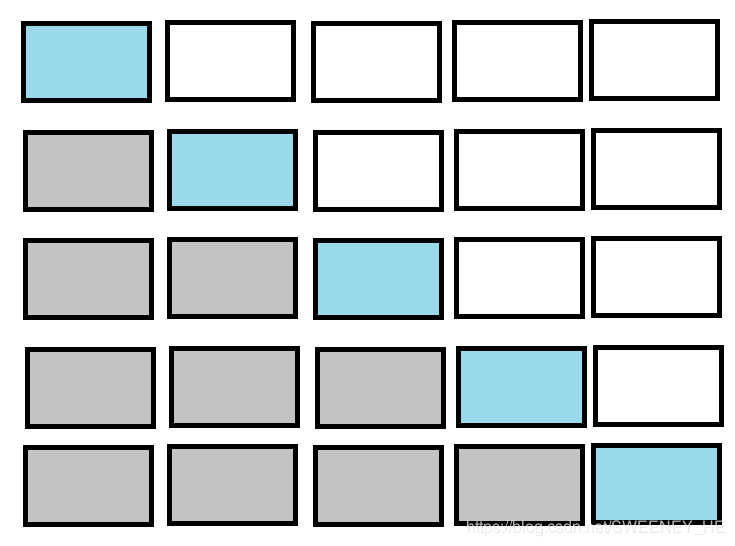二.压缩原理
根据上、下三角矩阵的特殊性(有一小半部分的元素都为一个常数C或者0)我们可以考虑将这一半的空间压缩到一个元素（多对一的映射)，然后另一半的部分就类似对称矩阵一半部分的压缩存储了。
三.矩阵坐标[i,j]转换为一维数组下标K的方法
1>下三角矩阵：
(1).首先考虑，非重复部分的存储，见图：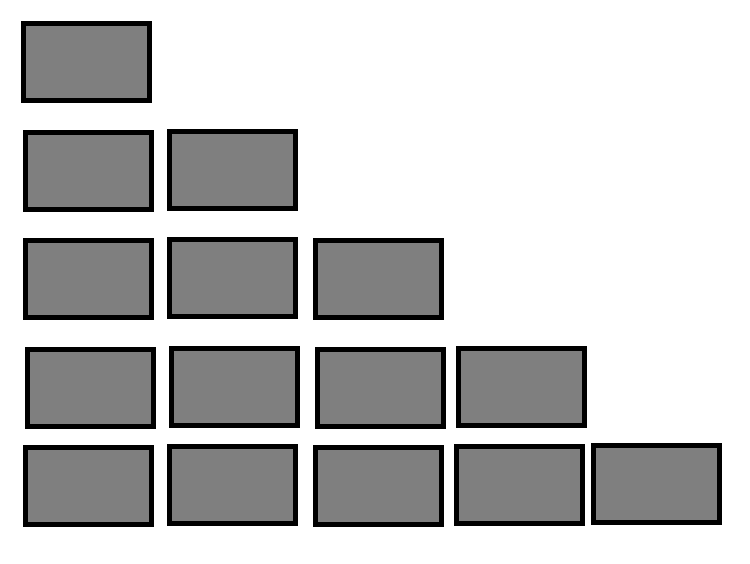可以得出k = i(i-1)/2+j-1 （推导过程可以参考：对称矩阵的压缩https://blog.csdn.net/SWEENEY_HE/article/details/85951555）
(2).重复部分只需一个元素即可，为不影响前面的存储，考虑放到所有非重复元素的后面即可， 由于前面部分总共的元素个数为：
n(1+n)/2(等差数列求和，每行元素逐级递增）又由于数组以0为起点，所以放到n(1+n)/2的位置即可。
总结：
k = i(i-1)/2+j-1 (i<=j)
k = n(n+1)/2 (i>j)
2>上三角矩阵
(1).先考虑非重复部分的存储，图示：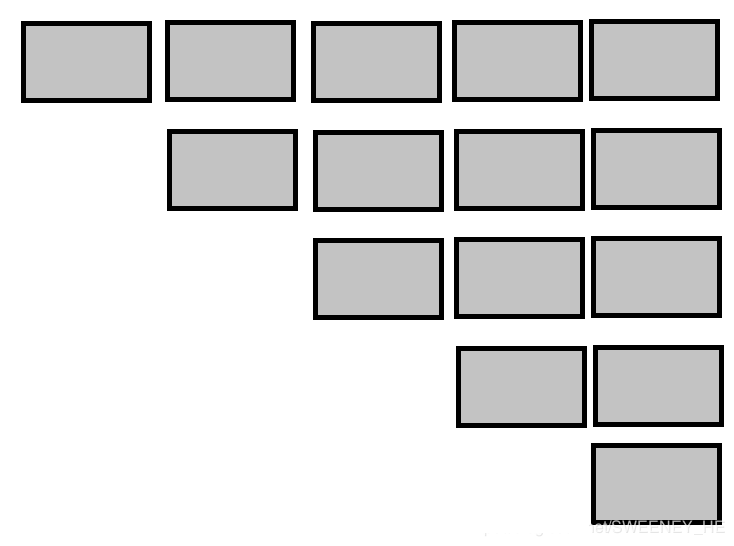按行优先存储，矩阵中元素对应一维数组的元素规则为：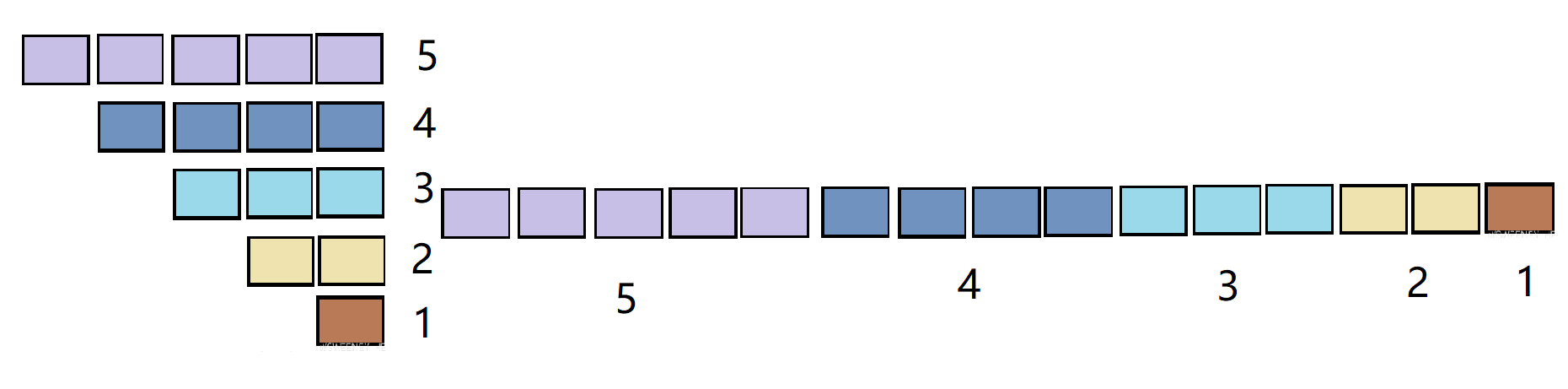由图显而易见，某元素在一维数组中的下标就是它按行优先存储时在半个矩阵中的序号
(1-1)序号 = 所有在它前面元素的个数 = 它所在行前面行的所有元素+它所在行它前面的元素
由于每行元素个数逐级递减，构成一个等差数列，公差：d = 1,首项：a1 = n ,末项：an = n-(i-1) = n-i+1（经评论区提醒，已自纠）
(1-2)前i-1行元素个数为：sn = n(a1+an)/2 = (i-1){n+[n-(i-1)+1]}/2=(i-1)(2n-i+2)/2
(1-3)第i行中在它前面的元素个数为：j-i  （元素逐行减少，可以看成从行首拿掉一个元素，所以第i行已经被拿走了i-1个元素，第i行的第j列元素前面就只有(j-1)-(i-1) = j-i个元素了）
(1-4)公式：K =(i-1)(2n-i+2)/2+j-i
(2).考虑重复部分元素和下三角一样：k = n(n+1)/2
总结：
K = (i-1)(2n-i+2)/2+j-i(i<=j)
K = n(n+1)/2 (i>j)
展开全文• 10阶上三角矩阵压缩存储时需存储的元素个数为( ) A.11 B.56 C.100 D.101
• 对称矩阵 n阶矩阵中任意一个元素aij都有aij=aji，则为对称矩阵 只存储主对角线+下三角区（按行优先存储在一维数组中） ...三角矩阵 三对角矩阵 稀疏矩阵 三元表（行，列，值） 十字链表 ...
对称矩阵

n阶矩阵中任意一个元素aij都有aij=aji，则为对称矩阵

只存储主对角线+下三角区（按行优先存储在一维数组中）
数组大小：(1+n)*n/2三角矩阵三对角矩阵稀疏矩阵
三元表（行，列，值）  十字链表展开全文数据结构
• 本篇博客会较为详细地讲一下我个人对三角矩阵压缩存储公式的理解，希望能给后面的朋友们带来一些帮助。
前言
本篇博客会较为详细地讲一下我个人对三角矩阵压缩存储公式的理解，希望能给后面的朋友们带来一些帮助。

等差数列的求和公式
由于三角矩阵的压缩存储公式是依靠求和公式来推导的，所以得先补一下等差数列的求和公式。
求和公式一：
其中n是整个数列的项数，是数列的首项，d是数列的公差（递增数列公差为正数，递减数列公差为负数）。

求和公式二：
其中n为整个数列的项数，是数列的首项，是数列的末项。下面主要用到这个公式二。

上三角矩阵压缩储存公式的推导
一些说明上的约定，有助于你更好地理解：
我们讨论的是压缩储存上三角矩阵，所以全文提到的行都是针对上三角块而言的，例如我说所在行，指的是这三个元素组成的行，不包括 和 。注意理解“前面i-1行”的意思，例如i=5，那指的就是前面4行，是第i行的前面4行的意思。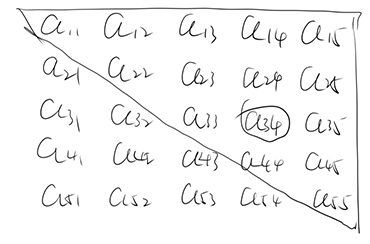首先我们知道，压缩储存上三角矩阵，本质上就是将矩阵的上三角块的元素“展开”成一条长的数列存在数组里。问题就在于，我们如何根据原矩阵里元素的行号和列号得到压缩后数组里对应的下标？
我们可以这样考虑：对于一个上三角块里第i行第j列的元素，它在数组里的下标就等于（在原矩阵中）第1到第i-1行的元素数量 + （原矩阵中）在他所在的行，他前面的元素数量。以下面这个矩阵为例，在数组里的位置就应该是它前面两行元素的数量5+4=9，再加上所在行它前面的元素数量1（即是这个元素），最终结果10即是在数组中的位置（当然，转换成物理下标的话还需要-1）。那么问题又来了，我们如何才能知道前面1到i-1行的元素数量？这个时候就要用到我们的等差数列求和公式了，我们可以从上到下地将每行的元素数量看成一个数列，对于上图的矩阵来说，这个数列就是5 4 3 2 1，项数为5，首项为5，末项为1，直接套进求和公式二，假设矩阵的维度n为5（意为5*5的矩阵），就可以得到，然后再加上用来存放下三角元素的空间1（上三角矩阵的下三角元素都为同一个常数，所以要花一个空间来存），得到，这就是压缩后数组大小的公式，其中n是矩阵的维度。
但这与我们要求第1行到第i-1行的元素数量有什么关系？确实没什么关系，上面这段是我补充的内容，但是理解了求整个数组大小的过程对后面有帮助，下面回到正题。
第1行到第i-1行的元素数量我们可以看成是一个数列，这个数列的首项是n（这个n也是矩阵的维度），末项是第i-1行的元素数量是n-i+2（找行号与该行的元素数量的规律可得，例如对来说，i是它的行号，即是5，那么它上一行的元素数量就是n-i+2，即是5 - 5 + 2 = 2个元素），数列的项数是i-1（从1到i就是有i项，从1到i-1自然就是有i-1项），套进求和公式二里可以得到，这样就解决了求第1行到第i-1行元素数量的问题。注意，这里数列的末项是指的上一行的元素数量，是i-1行的元素数量。
那么就到第二个问题，如何知道在所在行，前面的元素数量?看图，在所在行，前面有多少个元素？就是4 - 3 = 1个元素嘛；那所在行，前面有多少个元素？也是 5 - 3 = 2个元素，找一下规律就知道这个问题的答案是 j-i（列数减行数），那么我们最终的公式就出来了：
在数组里的位置=

嗯？好像与一些数据结构教材上给的公式对不上？这是因为我们的上图的矩阵元素是默认从开始的，所以上面那个最终公式我写的是在数组里的位置，而不是在数组里的下标。一般我们的矩阵行号列号都是从0开始算的，也就是（对上图矩阵而言）矩阵里第一个元素是最后一个元素是，那这样上面推导过程中出现的数组就变成是以n为首项，n-i+1为末项，项数为i的数列，套进求和公式二就可以得到，再加上该元素所在行，它前面的元素数量j-i，可得最终的公式：
在数组里的下标=
这个公式应该是和大部分数据结构教材给的公式一致的。

推导过程总结
因为推导过程写得比较乱，这里再总结一下，理清思路。
对于求元素在压缩后数组里下标的问题，我们可以转换思路，变成求前面有几个元素的问题；
求前面有几个元素的问题，我们又可以拆成求前面i-1行的元素数量的问题1和求在所在行，前面的元素数量的问题2；
对问题1，我们将矩阵每行的元素数量从上到下视为一个等差数列，利用等差数列的求和公式二求解。
对问题2，我们可以去找一下其中行列号的规律来解，也就是j-i，列数减行数。
推导过程中要注意的地方有三个：
数列的末项是对来说的i-1行，而不是所在的第i行。对第x行来说，元素的数量就等于n-x+1，其中n是原矩阵的维度，x是指逻辑上的顺序，从1开始。下面从0开始和从1开始的矩阵数列末项会不同也是因为x的取值不同所导致，公式不同也是。矩阵行列号从0还是从1开始的问题，从0开始的数列是一个首项为n，末项为n-i+1，项数为i的数列；从1开始的数列是一个首项为n，末项为n-i+2，项数为i-1的数列
最终公式是：
在数组里的下标=，其中i为行号j为列号，n为原矩阵维度

代码实现
#include <iostream>
#define dimSize 5 // dimension size

using namespace std;

typedef struct TriangularMatrix
{
int *data;
int dim;  // dimension of this matrix
TriangularMatrix() : data(NULL), dim(-1) {}
} Mat;

int getArraySize(Mat *mat)
{
int dim = mat->dim;
int sz = dim * (dim + 1) / 2 + 1; //+1 for saving constant C
return sz;
}

Mat *createMat()
{
Mat *mat = new Mat;
mat->dim = dimSize;
mat->data = new int[getArraySize(mat)]{0};
return mat;
}

void destroyMat(Mat *&mat)
{
delete[] mat->data;
delete mat;
mat = NULL;
}

bool checkIndex(Mat *mat, int col, int row)
{
int n = mat->dim;
if (col > n || row > n)
return false;
return true;
}

void printArr(Mat *mat)
{
int sz = getArraySize(mat);
cout << "TMat:[";
for (int i = 0; i < sz; i++)
{
cout << mat->data[i];
if (i != sz - 1)
cout << " ";
}
cout << "] " << sz << endl;
}

void initTMat(Mat *mat, int *data)
{
int n = mat->dim;
int k = 0;
int tmp;
for (int i = 0; i < n; i++) //row
{
for (int j = i; j < n; j++) //column
{
if (j >= i)
{
tmp = i * n + j;
mat->data[k++] = data[tmp];
}
}
}
mat->data[k] = data[(n - 1) + 1]; //constant C
}

int getValue(Mat *mat, int col, int row) // col and row are logical indices (based-1)
{
if (!checkIndex(mat, col, row))
return INT_MIN;
int n = mat->dim;
--col; //logical index turn to physical index
--row; //ditto
if (col >= row)
return mat->data[row * (2 * n - row + 1) / 2 + col - row];
else //if col < row
return mat->data[getArraySize(mat) - 1];
}

void printTMat(Mat *mat)
{
int n = mat->dim;
for (int r = 1; r <= n; r++) //row
{
for (int c = 1; c <= n; c++) //column
cout << getValue(mat, c, r) << " ";
cout << endl;
}
}

int main()
{
Mat *mat = createMat();

int arr{
0, 1, 2, 3, 4,
0, 5, 6, 7, 8,
0, 0, 9, 10, 11,
0, 0, 0, 12, 13,
0, 0, 0, 0, 14};
initTMat(mat, arr);
printArr(mat);
printTMat(mat);
destroyMat(mat);
return 0;
}
在重点地方都给了英文的注释，这里再补充几点：
开数组的时候给多了一个空间是用来储存常数，因为对于上三角矩阵来说，下三角块就是一个常数，也需要一个数组空间来存。initTMat里用了上三角矩阵的性质来用if筛选元素存进数组里；第二个for的初始化语句int j = i是灵光一闪想到的优化循环次数的条件；最后一句取下三角常数的语句是随便挑的，顺带考虑了一下普适性用了维度作为下标，如果取一个特定值的话可能在更大维度的矩阵上会取到上三角的值。getValue的参数行号和列号是逻辑的，也就是从1开始的，代码里用自减转换为物理下标。

本文到此结束。


展开全文上三角矩阵 数据结构
• 线性压缩存储三角矩阵三角矩阵： 上三角矩阵： 以下三角矩阵的线性压缩存储为例，进行实现： package pers.zhang.array; /** * @author zhang * @date 2020/1/19 - 13:34 * * 下三角矩阵线性压缩存储 ...数据结构
• 输出原来的矩阵；输出压缩后的一维数组；根据输入的行号列号，从压缩矩阵中计算出元素的值 #include<stdio.h> int main(){ inta={ //定义原二维数组 1,0, 0, 0, 0, 5,9, 0, 0, 0, 4,6,...压缩存储
• 矩阵的压缩存储–特殊矩阵–三角矩阵三角矩阵大体分为三类：对称矩阵、下三角矩阵和上三角矩阵。#include #include #define N 100 int a[N][N], sa[2*N]; int main(void) { int i, j, k; int n, m; printf("请...数据结构 对称矩阵
•数据结构 c++
• 上、下三角压缩存储和对称矩阵压缩存储（上三角部分、下三角部分）类似，不过是多了一个常数要存储。 对称矩阵压缩存储   代码 #include <iostream> using namespace std; int main(){ int n; ...
• 4.2 矩阵的压缩存储（二） 4.2.1特殊矩阵 2.三角矩阵 以主对角线划分，三角矩阵有上三角和下三角两种。下三角矩阵正好相反，它的主对角线上方均为常数c或零；上三角矩阵是指矩阵的下三角（不包括对角线）中的元素均...数据结构
• 三角矩阵：|i-j|<=1的位置上为非零元素，也就是我们要存储的元素；|i-j|>1的位置上全是0，对于0我们不进行压缩存储。 原理： 矩阵从下标1开始，对于ai,j，其前i-1行共有3*(i-1)-1个元素（第一行为2个，其余...
• 已知矩阵A是一个下三角矩阵，如下图 要求编写算法把矩阵A采用压缩存储，存储到一维数组B中， 并且依次输出B中各元素的值以验证该算法功能已实现 */ #include using namespace std; const int m=5; const ...算法 system c
• #include #include #define N 4//为N阶对称矩阵初始化存储数据的一维数组Bvoid Init(int *&b){b = (int*)malloc(sizeof(int)*(N*(N+1)/2));}//返回存储在b[M]中，对应二维数组A[i][j]的值int Value(int b[], int...
• 对称矩阵压缩实现原理c二维数组存储在c中矩阵的表示是用二维数组。那么首先要搞清楚数组行列与矩阵行列的对应。在c语言中二维数组是按行存储的。即顺序存储每一行。（第一行，第二行。。。最后一行） 看一下例子...二维数组
• // 对称矩阵的输入 void Symmetric_input(int **A, int n, int B[]) { int i, j, k = 0; // 将对称元素及对称轴上的元素存入数组B for (i = 0; i < n; i++) for (j = 0; j < i + 1; j++)c语言 算法 数组
• 对称矩阵及对称矩阵压缩存储设一个N*N的方阵A，A中任意元素Aij，当且仅当Aij == Aji(0 <...压缩存储矩阵存储时只需要存储上三角/下三角的数据，所以最多存储n(n+1)/2个数据。对称矩阵压缩存储的对应...
• ## 矩阵压缩存储思路

千次阅读 2016-09-12 22:58:07
特殊矩阵主要包括 n阶对称矩阵 上三角矩阵（下三角矩阵） 稀疏矩阵 n阶对称矩阵的条件如下： a[i][j]=a[j][i] 1 因为是把二维数组存入一维数组，所以二维数组的下标肯定跟一维数组存在某种关系（稀疏矩阵除外） ...
• 对称矩阵存储： 代码如下: #include <iostream> using namespace std; int main() { int n; cin >> n; int *a; a = new int[(n*(n + 1)) / 2]; for (int i = 0; i < (n*(n + 1)) / 2; i++) ...数据结构 算法 c++ 数组
• 对于数组的压缩存储，一维数组主要使用哈夫曼压缩，多维数组主要采用矩阵压缩的形式，对特殊矩阵和系数矩阵进行压缩。 哈夫曼压缩 哈夫曼压缩是由哈夫曼树推广而来的，是哈夫曼编码的重要应用。哈夫曼树 ─ 即最优...哈夫曼...